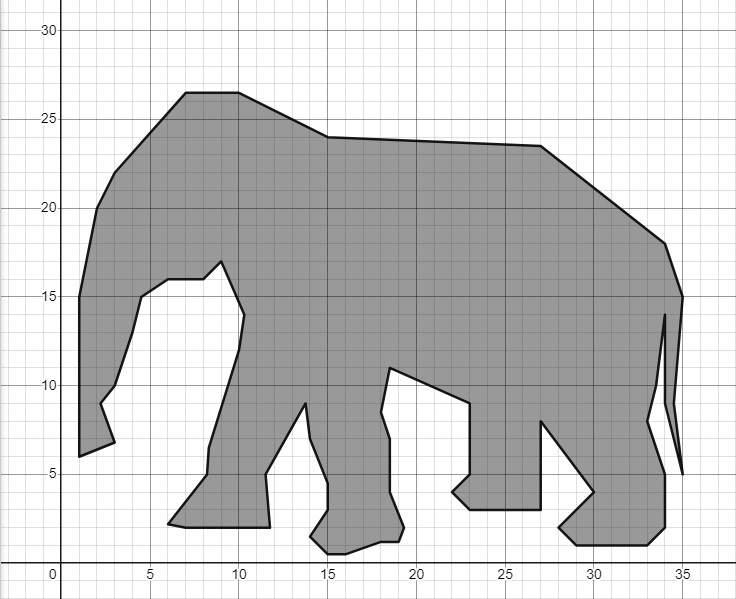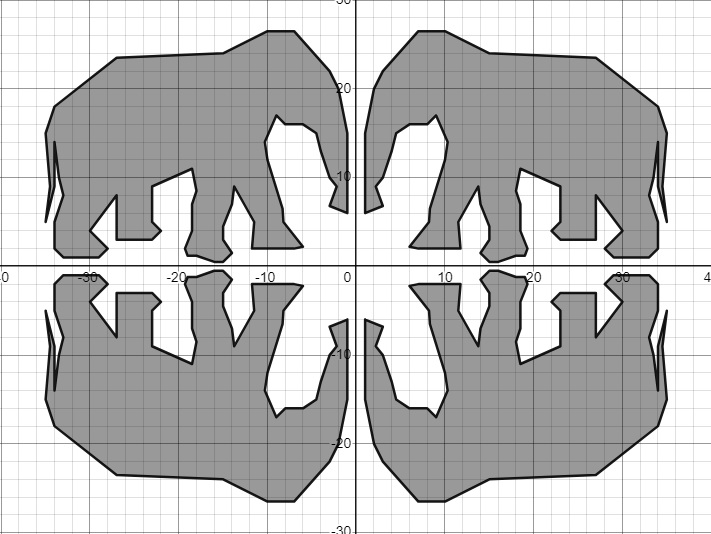# 1766 A Polygonal Elephant for World Elephant Day

Contents

### Today’s Puzzle:

Today many people are reflecting on the plight of elephants throughout the world and what we can do to protect them and their habitats. Yesterday I created an image of a lone elephant in Desmos:Today’s puzzle is to take the Desmos Elephant image and transform it as I have done below. There is no need to retype all the ordered pairs that made the elephant. One of the reflections was made simply by typing “polygon(x1,-y1)” below all the order pairs in the Desmos image. Can you determine which reflection that was? What was typed inside the parenthesis to make the other two elephants?How would the elephant be transformed if you typed “polygon(-y1,x1)”, “polygon(x1+5,y1)” or  “polygon(2×1,2y1)” below the ordered pairs in Desmos? Can you figure out a way to shrink the elephant and make it upside down in the first quadrant? I hope you will take the time to check out these transformations and experiment with some of your own.

### Factors of 1766:

• 1766 is a composite number.
• Prime factorization: 1766 = 2 × 883.
• 1766 has no exponents greater than 1 in its prime factorization, so √1766 cannot be simplified.
• The exponents in the prime factorization are 1 and 1. Adding one to each exponent and multiplying we get (1 + 1)(1 + 1) = 2 × 2 = 4. Therefore 1766 has exactly 4 factors.
• The factors of 1766 are outlined with their factor pair partners in the graphic below.### More About the Number 1766:

1766 looks interesting in some other bases:
It’s 6E6 in base 16 because 6(16²) + 14(16) + 6(1) = 1766,
272 in base 28 because 2(28²) + 7(28) + 2(1) = 1766, and
123 in base 41 because 1(41²) + 2(41) + 3(1) = 1766.

This site uses Akismet to reduce spam. Learn how your comment data is processed.